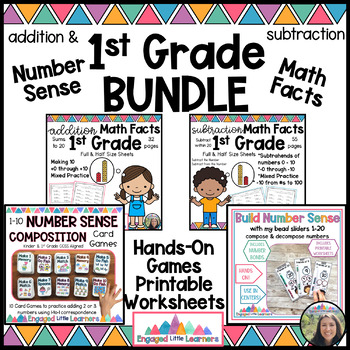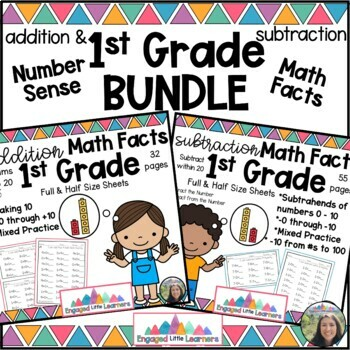DID YOU KNOW:
Seamlessly assign resources as digital activities

Learn how in 5 minutes with a tutorial resource. Try it Now

Learn More# Math Facts for 1st Grade : Build Number Sense: Addition & Subtraction BUNDLEK - 1st
Subjects
Standards
Resource Type
Formats Included
• Zip
• Compatible withActivities
Pages
188 pages
\$6.50
Bundle
List Price:
\$9.25
You Save:
\$2.75
\$6.50
Bundle
List Price:
\$9.25
You Save:
\$2.75
Compatible with Easel Activities
Some resources in this bundle can be made into interactive versions that students can complete on any device. Easel is free to use! Learn more.

#### Also included in

1. This MEGA BUNDLE is a must have to help your 1st and 2nd graders develop an understanding of number sense & become fluent with the math facts to 20!The included products are:>> My BALLOON MATH Fact Addition Fluency Program (a \$38.00 Value) This is a complete program to teach your 1st an
\$25.00
\$50.00
Save \$25.00

### Description

This Bundle includes 4 products that are Common Core Aligned for 1st Graders! The following 4 products are to help you Build Number Sense, Addition & Subtraction Facts within 10.

1. Bead Sliders will give your students a hands-on tool to help them understand number combinations within 10. This will help build their Number Sense!

3. Addition Math Fact Fluency Practice Pages

4. Subtraction Math Fact Fluency Practice Pages

If you are interested in adding some more fun and motivation to your math fact routine, then check out Balloon Math! It is FULL of everything you need to teach addition facts for 1st & 2nd graders!

Total Pages
188 pages
N/A
Teaching Duration
N/A
Report this Resource to TpT
Reported resources will be reviewed by our team. Report this resource to let us know if this resource violates TpT’s content guidelines.

### Standards

to see state-specific standards (only available in the US).
Determine the unknown whole number in an addition or subtraction equation relating three whole numbers. For example, determine the unknown number that makes the equation true in each of the equations 8 + ? = 11, 5 = ▯ - 3, 6 + 6 = ▯.
Add and subtract within 20, demonstrating fluency for addition and subtraction within 10. Use strategies such as counting on; making ten (e.g., 8 + 6 = 8 + 2 + 4 = 10 + 4 = 14); decomposing a number leading to a ten (e.g., 13 - 4 = 13 - 3 - 1 = 10 - 1 = 9); using the relationship between addition and subtraction (e.g., knowing that 8 + 4 = 12, one knows 12 - 8 = 4); and creating equivalent but easier or known sums (e.g., adding 6 + 7 by creating the known equivalent 6 + 6 + 1 = 12 + 1 = 13).
Relate counting to addition and subtraction (e.g., by counting on 2 to add 2).
Understand subtraction as an unknown-addend problem. For example, subtract 10 – 8 by finding the number that makes 10 when added to 8.
Apply properties of operations as strategies to add and subtract. If 8 + 3 = 11 is known, then 3 + 8 = 11 is also known. (Commutative property of addition.) To add 2 + 6 + 4, the second two numbers can be added to make a ten, so 2 + 6 + 4 = 2 + 10 = 12. (Associative property of addition.)